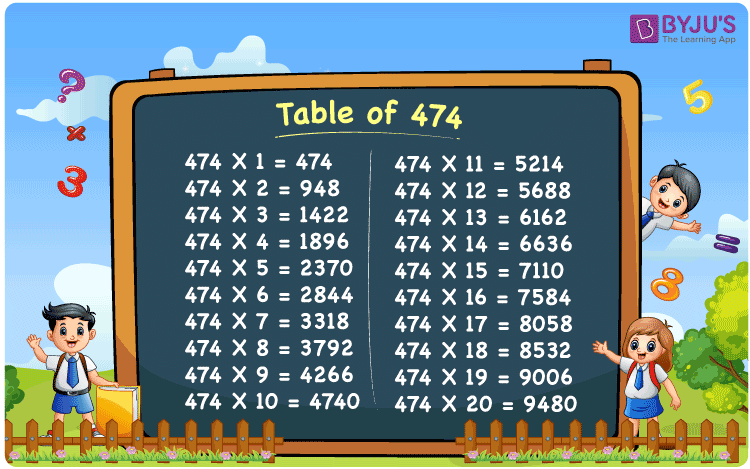Checkout JEE MAINS 2022 Question Paper Analysis : Checkout JEE MAINS 2022 Question Paper Analysis :

# Table of 474

The Table of 474 is given here to help students quickly check the values of the 474 times table up to 20. The multiplication table of 474 is given below in a tabular format, and a PDF is also provided to download. Students can download the printable PDF and use the image as a flashcard, which will be beneficial for memorising the 474 times table.

## Table of 474 Chart

The table chart of 474 would be helpful for a quick check with the multiples of 474. The printable image for the table of 474 can be used as a flashcard, and is valuable for doing arithmetic calculations, and solving problems for competitive exams.## What is the 474 Times Table?

The 474 times table is the multiplication of 474 with any natural number. The 474 times table can be obtained both by multiplication and by adding 474 repeatedly. The below chart shows the multiplication table of 474, in both multiplication and addition form.

 474×1 = 474 474 474×2 = 948 474 + 474 = 948 474×3 = 1422 474 + 474 + 474 = 1422 474×4 = 1896 474 + 474 + 474 + 474 = 1896 474×5 = 2370 474 + 474 + 474 + 474 + 474 = 2370 474×6 = 2844 474 + 474 + 474 + 474 + 474 + 474 = 2844 474×7 = 3318 474 + 474 + 474 + 474 + 474 + 474 + 474 = 3318 474×8 = 3792 474 + 474 + 474 + 474 + 474 + 474 + 474 + 474 = 3792 474×9 = 4266 474 + 474 + 474 + 474 + 474 + 474 + 474 + 474 + 474 = 4266 474×10 = 4740 474 + 474 + 474 + 474 + 474 + 474 + 474 + 474 + 474 + 474 = 4740

## Multiplication Table of 474

Given below are the first 20 multiples of 474.

 474 × 1 = 474 474 × 2 = 948 474 × 3 = 1422 474 × 4 = 1896 474 × 5 = 2370 474 × 6 = 2844 474 × 7 = 3318 474 × 8 = 3792 474 × 9 = 4266 474 × 10 = 4740 474 × 11 = 5214 474 × 12 = 5688 474 × 13 = 6162 474 × 14 = 6636 474 × 15 = 7110 474 × 16 = 7584 474 × 17 = 8058 474 × 18 = 8532 474 × 19 = 9006 474 × 20 = 9480

## Solved Example on the Table of 474

Example 1:

What is the sum of 8 times 474 and 2 times 474?

Solution:

8 times 474 = 474 × 8 = 3792

2 times 474 = 474 × 2 = 948

Therefore, 3792 + 948 = 4740.

## Frequently Asked Questions on the Table of 474

### What is the 474 times table?

The 474 times table is the multiplication table of 474 with natural numbers. For example, 474 × 1 = 474, 474 × 2 = 272, 474 × 3 = 408 and so on.

### What times table has 474?

474 comes in the times tables of 1, 2, 4, 8, 17, 34, 68 and 474.

### What is 33 times 474?

33 times 474 is 15,642.